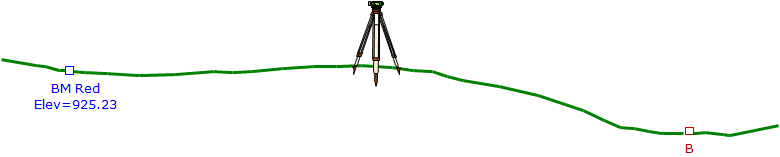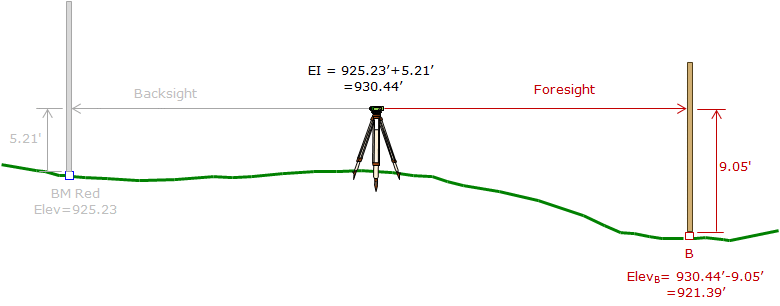## 1. Principle

Differential leveling uses the vertical distance difference between two points to transfer an elevation from one point to another.

A summary of the process:

A Level is setup between two points, one whose elevation is known, Figure B-1.Figure B-1 Setting Up

A backsight (BS) reading is taken on the known point, Figure B-2, using a rod divided into feet (or meters) and subdivisions thereof. This determines how far above the LoS is above the known point. Adding the BS reading to the point elevation gives the elevation of the instrument (EI, aka, Height of the Instrument, HI).Figure B-2 Backsight Reading

A foresight (FS) reading is taken on the unknown point, Figure B-3, to determine how far above it the LoS is. Subtracting the FS reading from the instrument elevation gives the point elevation.Figure B-3 Foresight Reading

The elevation of B is determined by adding the difference between the BS and FS readings:

ElevB = ElevRed+(BSRed-FSB)

The instrument is moved to a position between B and the next point and the process repeated.